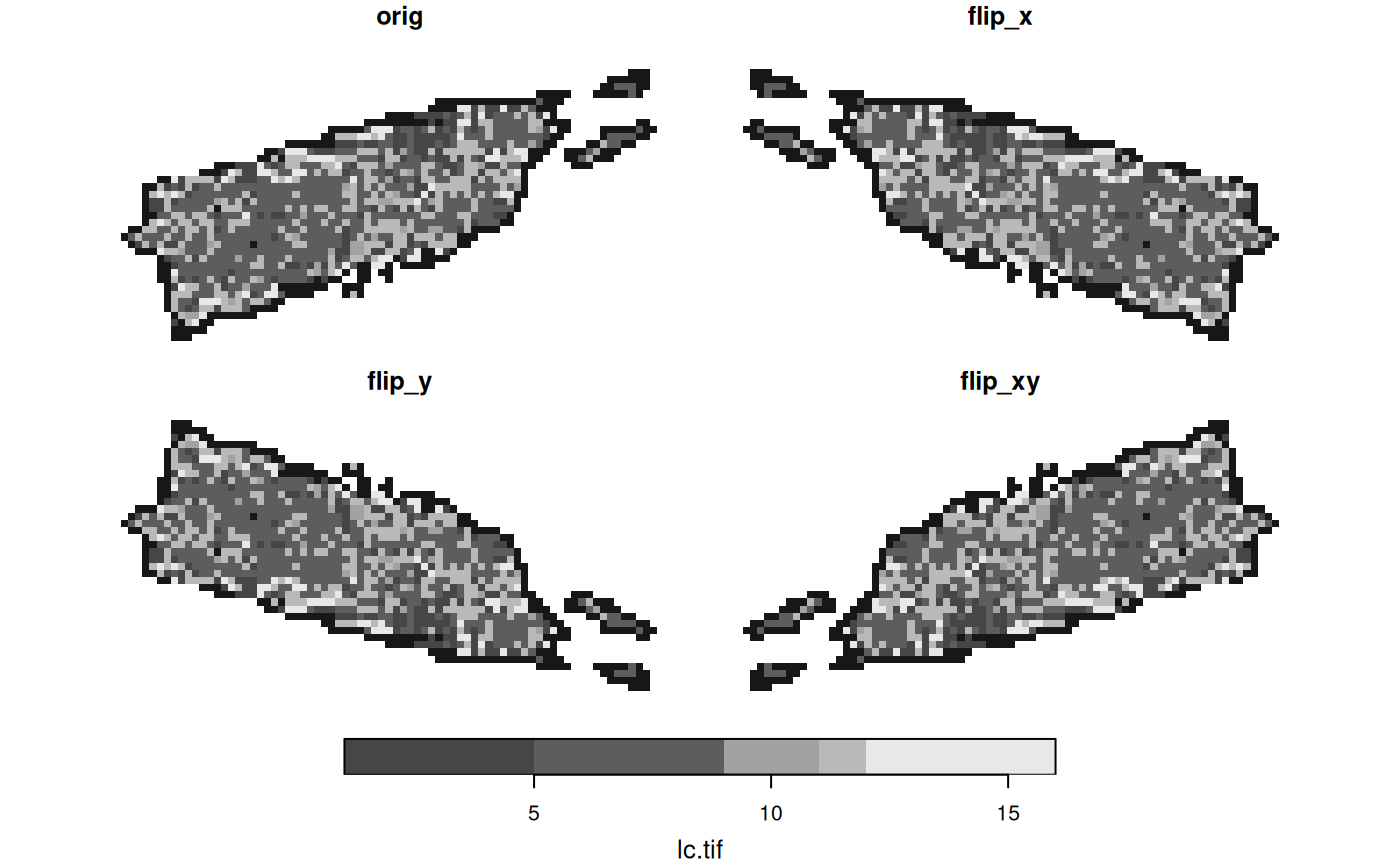subset stars objects

# S3 method for stars
[(x, i = TRUE, ..., drop = FALSE, crop = !is_curvilinear(x))

# S3 method for stars
[(x, i, downsample = 0) <- value

st_flip(x, which = 1)

## Arguments

x

object of class stars

i

first selector: integer, logical or character vector indicating attributes to select, or object of class sf or sfc used as spatial selector; see details

...

further (logical or integer vector) selectors, matched by order, to select on individual dimensions

drop

logical; if TRUE, degenerate dimensions (with only one value) are dropped

crop

logical; if TRUE and parameter i is a spatial geometry (sf or sfc) object, the extent (bounding box) of the result is cropped to match the extent of i using st_crop. Cropping curvilinear grids is not supported.

downsample

downsampling rate used in case i is a stars_proxy object

value

array of dimensions equal to those in x, or a vector or value that will be recycled to such an array

which

character or integer; dimension(s) to be flipped

## Value

st_flip flips (reverts) the array values along the chosen dimension without(s) changing the dimension properties

## Details

if i is an object of class sf, sfc or bbox, the spatial subset covering this geometry is selected, possibly followed by cropping the extent. Array values for which the cell centre is not inside the geometry are assigned NA.

in an assignment (or replacement form, [<-), argument i needs to be a stars object with dimensions identical to x, and value will be recycled to the dimensions of the arrays in x.

## Examples

tif = system.file("tif/L7_ETMs.tif", package = "stars")
x[,,,1:3] # select bands
#> stars object with 3 dimensions and 1 attribute
#> attribute(s):
#>              Min. 1st Qu. Median     Mean 3rd Qu. Max.
#> L7_ETMs.tif    21      58     70 70.36041      83  255
#> dimension(s):
#>      from  to  offset delta                     refsys point values x/y
#> x       1 349  288776  28.5 SIRGAS 2000 / UTM zone 25S FALSE   NULL [x]
#> y       1 352 9120761 -28.5 SIRGAS 2000 / UTM zone 25S FALSE   NULL [y]
#> band    1   3      NA    NA                         NA    NA   NULL
x[,1:100,100:200,] # select x and y by range
#> stars object with 3 dimensions and 1 attribute
#> attribute(s):
#>              Min. 1st Qu. Median     Mean 3rd Qu. Max.
#> L7_ETMs.tif    13      54     65 67.21531      77  252
#> dimension(s):
#>      from  to  offset delta                     refsys point values x/y
#> x       1 100  288776  28.5 SIRGAS 2000 / UTM zone 25S FALSE   NULL [x]
#> y     100 200 9120761 -28.5 SIRGAS 2000 / UTM zone 25S FALSE   NULL [y]
#> band    1   6      NA    NA                         NA    NA   NULL
x["L7_ETMs.tif"] # select attribute
#> stars object with 3 dimensions and 1 attribute
#> attribute(s):
#>              Min. 1st Qu. Median     Mean 3rd Qu. Max.
#> L7_ETMs.tif     1      54     69 68.91242      86  255
#> dimension(s):
#>      from  to  offset delta                     refsys point values x/y
#> x       1 349  288776  28.5 SIRGAS 2000 / UTM zone 25S FALSE   NULL [x]
#> y       1 352 9120761 -28.5 SIRGAS 2000 / UTM zone 25S FALSE   NULL [y]
#> band    1   6      NA    NA                         NA    NA   NULL
xy = structure(list(x = c(293253.999046018, 296400.196497684), y = c(9113801.64775462,
9111328.49619133)), .Names = c("x", "y"))
pts = st_as_sf(data.frame(do.call(cbind, xy)), coords = c("x", "y"), crs = st_crs(x))
image(x, axes = TRUE)
plot(st_as_sfc(st_bbox(pts)), col = NA, add = TRUE)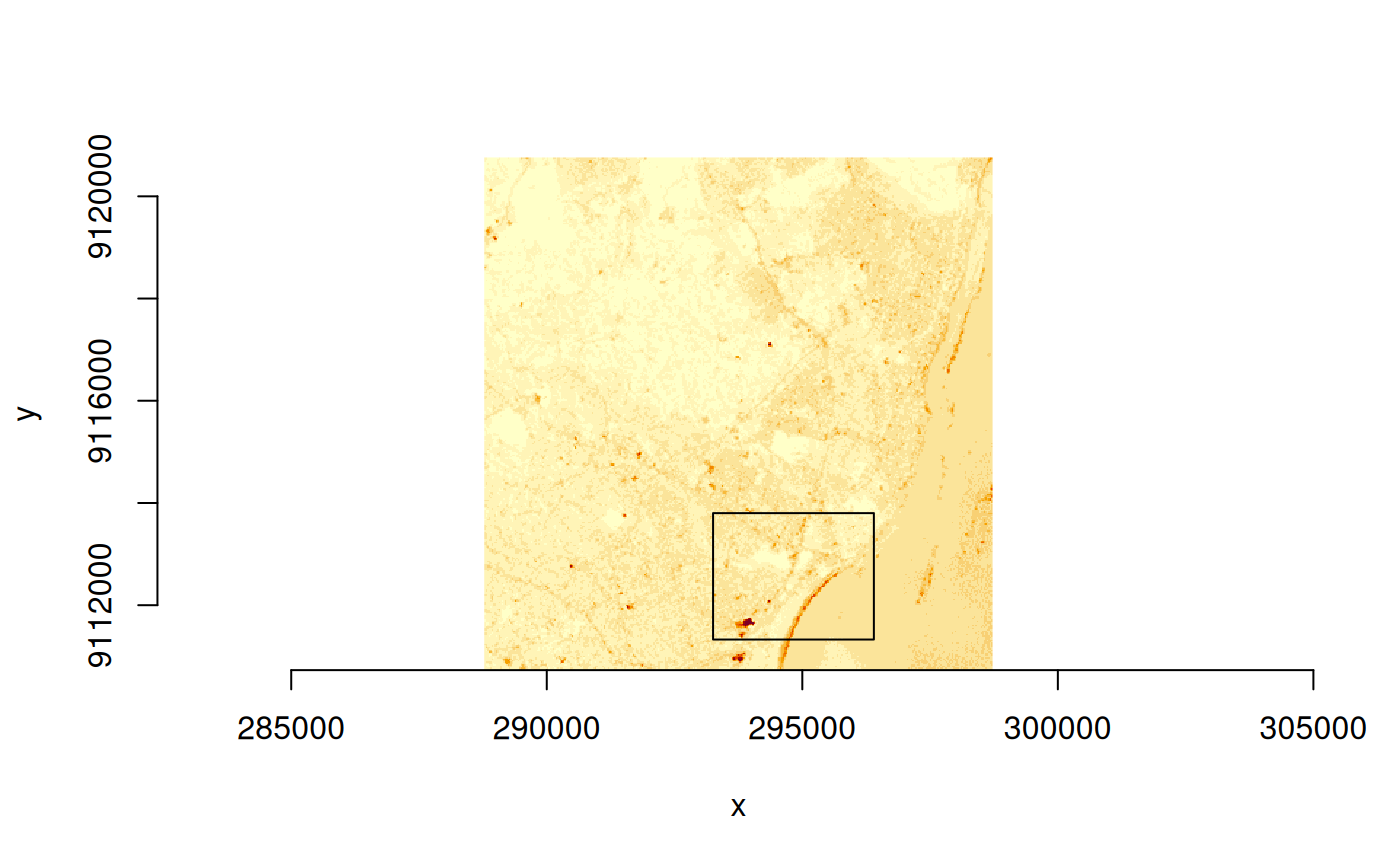bb = st_bbox(pts)
(xx = x[bb])
#> stars object with 3 dimensions and 1 attribute
#> attribute(s):
#>              Min. 1st Qu. Median     Mean 3rd Qu. Max.
#> L7_ETMs.tif     3      56     73 70.87303      89  255
#> dimension(s):
#>      from  to  offset delta                     refsys point values x/y
#> x     158 268  288776  28.5 SIRGAS 2000 / UTM zone 25S FALSE   NULL [x]
#> y     245 331 9120761 -28.5 SIRGAS 2000 / UTM zone 25S FALSE   NULL [y]
#> band    1   6      NA    NA                         NA    NA   NULL
image(xx)
plot(st_as_sfc(bb), add = TRUE, col = NA)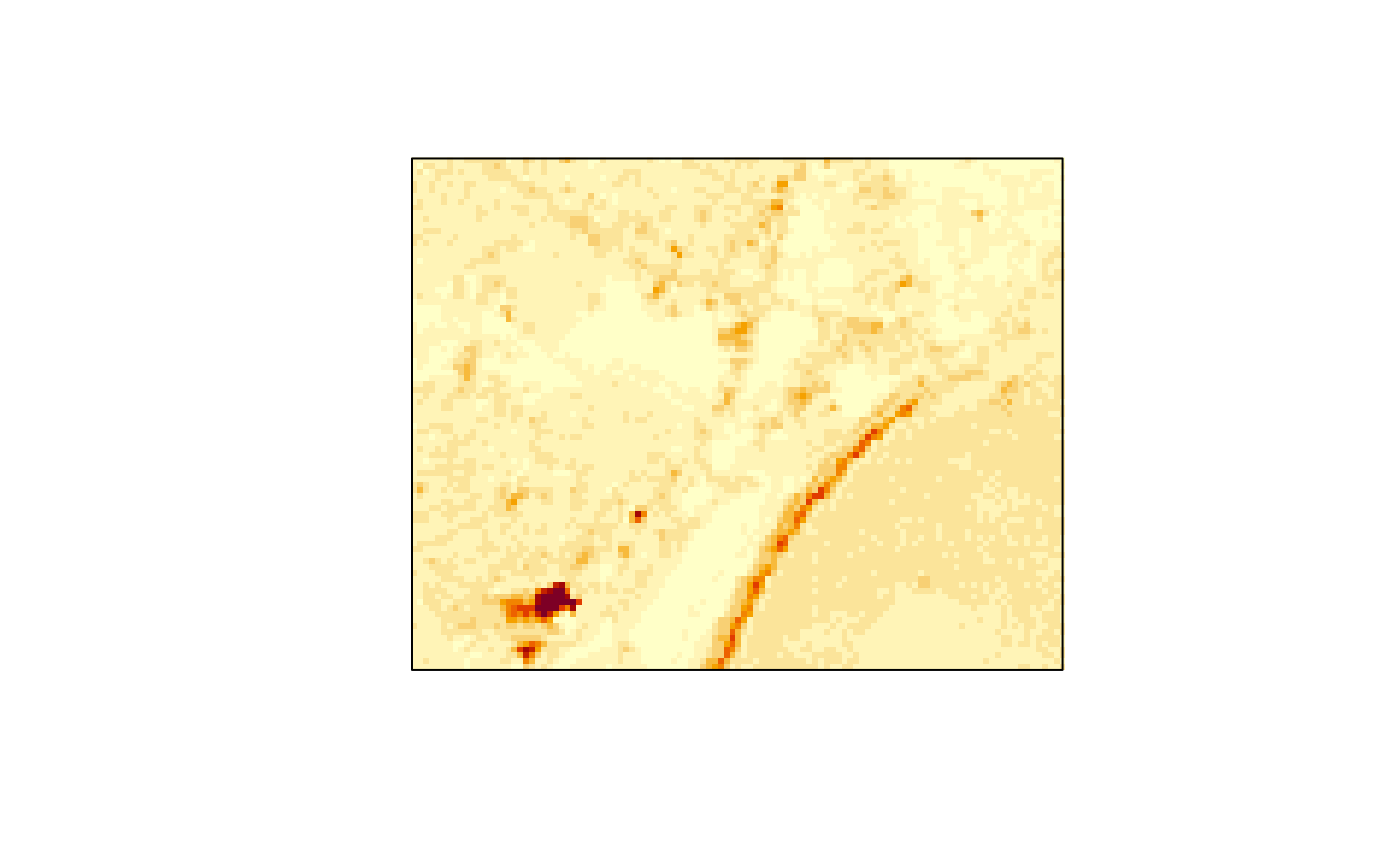image(x)
pt = st_point(c(x = 290462.103109179, y = 9114202.32594085))
buf = st_buffer(st_sfc(pt, crs = st_crs(x)), 1500)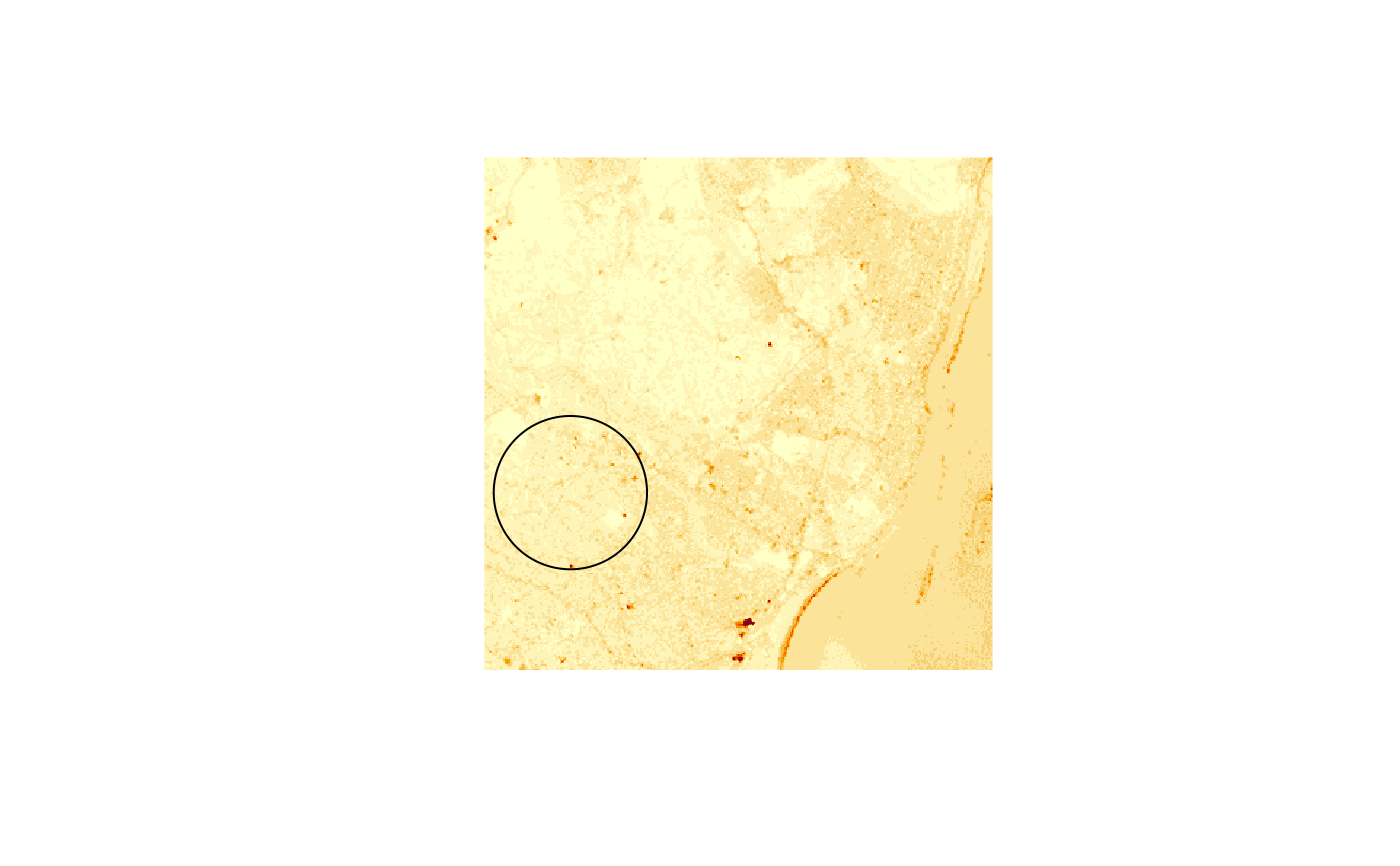buf = st_sfc(st_polygon(list(st_buffer(pt, 1500)[], st_buffer(pt, 1000)[])),
crs = st_crs(x))
image(x[buf])
plot(buf, add = TRUE, col = NA)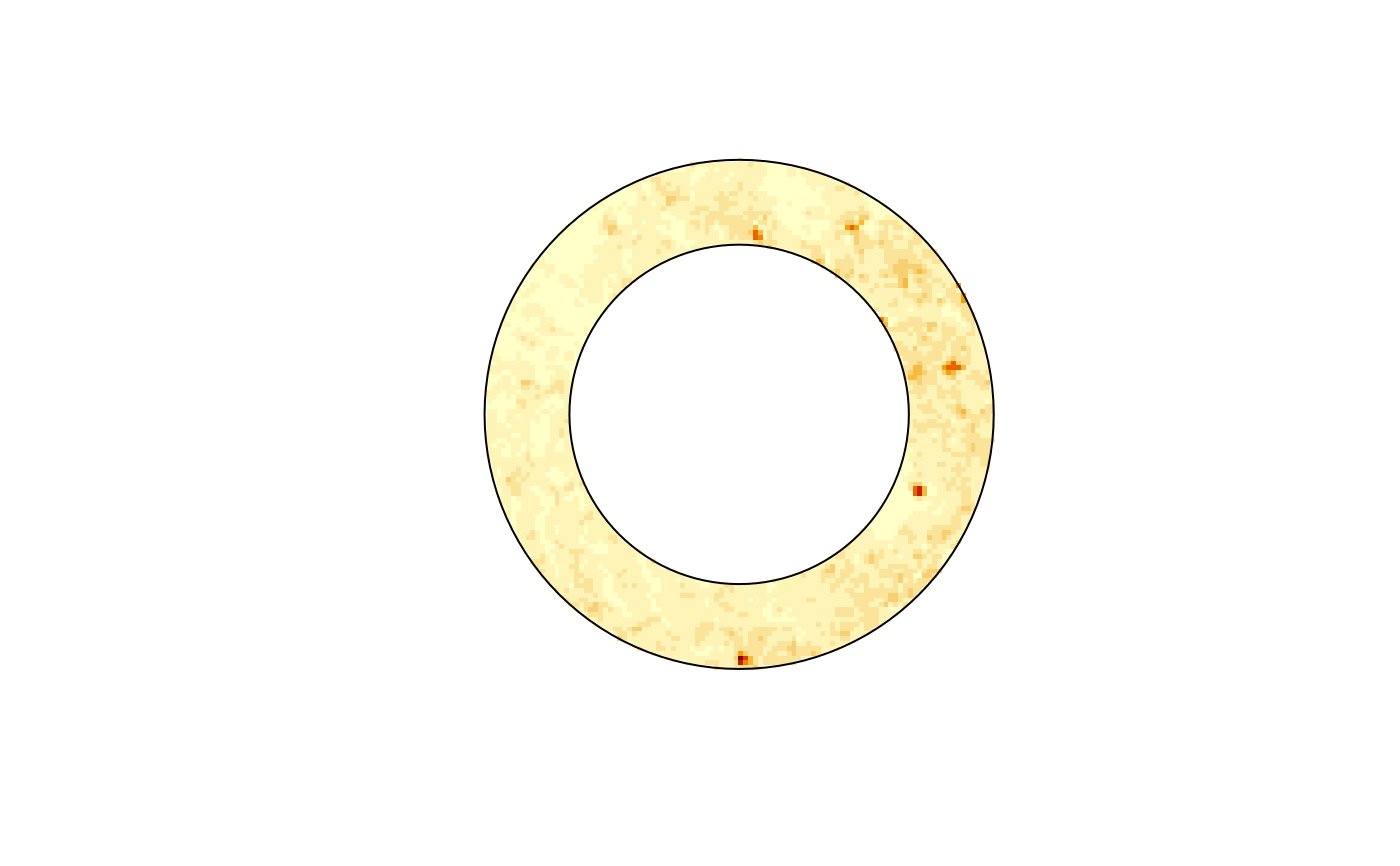image(x[buf, crop=FALSE])
plot(buf, add = TRUE, col = NA)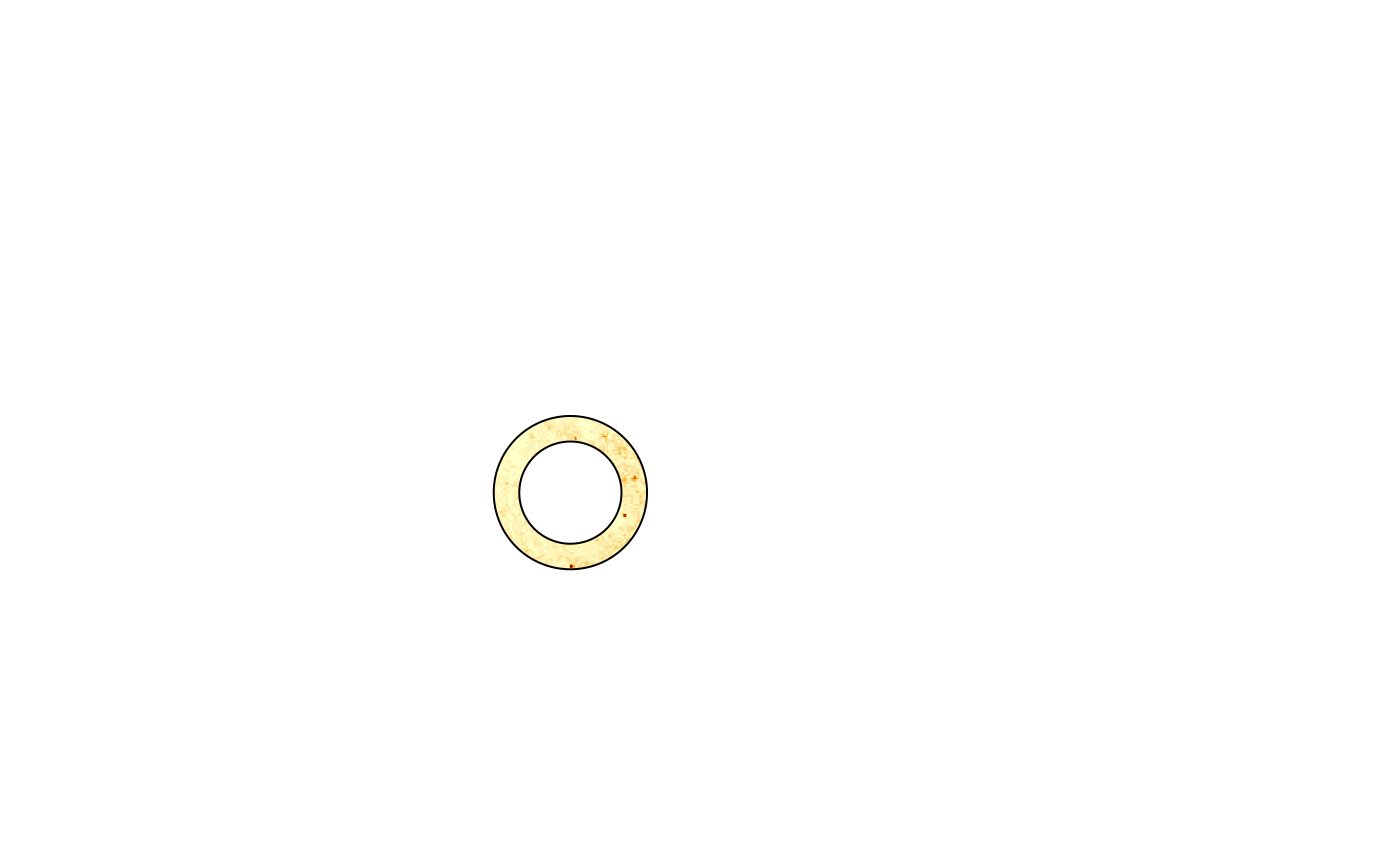lc = read_stars(system.file("tif/lc.tif", package = "stars"))
#> Warning: categorical data values starting at 0 are shifted with one to start at 1
x = c(orig = lc,
flip_x = st_flip(lc, "x"),
flip_y = st_flip(lc, "y"),
flip_xy = st_flip(lc, c("x", "y")),
along = 3)
plot(x)